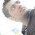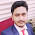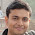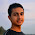## Feb 3, 2013

### Probability Puzzle: Guess Position of Card

Source: Counter-intuitive ConundrumsProblem:
Someone hands you a deck of cards which you thoroughly shuffle. Next, you start to deal them, face-up, counting the cards as you go. “One, Two, Three …”

The aim is to predict what the count will be when you encounter the second black Ace in the deck.

If you had to select one position before you started to deal, what number would you select that maximizes your chance of guessing the location of the second black Ace?

#### 13 comments:

1.last
n^th position probability = 2*(n-1)/((52)(51)) , maximizes at n=52

2.This comment has been removed by a blog administrator.

3.Last,
The problem can be thought of picking the first Ace from the desk upside down.
Now:
Prob Of 1st card being first black ace I pick = 2/52
Prob Of 2nd card being first black ace I pick = (50/52)*(2/51)
Prob Of 3rd card being first black ace I pick = (50/52)*(49/51)*(2/50)

As you see.. this sequence is decreasing, with max prob at starting, so prob is max at first card (for upside down deck)
Hence our prob is max for 52nd card !

4.Let the 2nd black ace be picked in the n-th turn, n>=2
A: The first black ace is picked in n-1 turns
B: The second black ace is picked in the n-th turn
P(A) = 2 * 50C(n-2) / 52C(n-1) = 2*(53-n)*(n-1)/(52*51)
P(B) = P(B|A) * P(A) = 1/(52-(n-1)) * P(A) = 2(n-1)/(52*51)
P(B) is max for n = 52

5.Expected count when second black ace appears
= (2/52)*(1/51)*2 + 2*(2/52)*(50/51)*(1/50)*3 + 3*(2/52)*(50/51)*(49/50)*(1/49)*4 + ....
= (2/52)*(1/51)*{2*1 + 3*2 + 4*3 + .... + 52*51}
= 35.333

Hence, expected count when one encounters the second black Ace in the deck = 35

6.18

On the 18th card , Probability of having 2nd ace is highest.
P(2nd ace is at xth place) = 4C2 * 2! * (x-1) * 48C(x-2) * (x-2)! * (52-x)! / 52!

This comes out to be c(x-1)(51-x)(52-x)
Maximizing it for integral x will give x =18

Also, On an average 2nd ace appears at 21.2 position.
48/5 * 2 + 1 + 1 = 21.2 (48 cards dividing into 5 equal blocks, so after 9.6 cards, 1st ace will appear, after another 9.6 cards another ace will appear).

7.answer is 52 as the probability of finding second black ace at k-th position is 2*(k-1)/(51*52).. it is maximized at k=52.

8.I used the following MATLAB code, and the answer turns out to be 35 (expected position ~35.36)

sum=0;
for N = 1:1:1e6
count=0;i=0;
A=randperm(52); % random permutation of integers from 1 to 52
while(count < 2)
i=i+1;
if mod(A(i),26)==0 % only 2 numbers in the set satisfy this condition
count = count+1;
end
end
sum = sum + i;
end

average = sum/N

9.@jeeudr. You are making a mistake by taking average. Consider a case where pos 40 has probability 0.5 and pos 42 has probability 0.5 , average gives pos as 41 where the probability is in fact 0

Better approach would be to keep a count for each position. Whichever position has higher count has higher probility

10.I wrote a python program to simulate this, the code is here https://github.com/sooriravindra/2nd_black_ace/blob/master/compare.py

I am also comparing the simulated case with theoretic case i.e, 2*x/(51*52)
The results agree with each other

11.Here is a play of words. The question is not asking the expected position of the second black ace (which will be infact 35, by using principle of symmetry).
It is asking the position, where you can find the second black ace with maximum probability. So, it should be 52.
Had it been the case that the numbers one, two, three, ... Follow normal distribution then both the answers would have been same. Normal distribution is just one example.

1.please explain how you arrived at expectation = 35 and how are you using principle of symmetry.

12.If we compute the expectation it comes out to be summation of (2*n^2-2*n)/51*52 when we put N= 52 we get the expectation to be 17.6

### Fraction Brainteaser

Source: Sent to me by Gaurav Sinha Problem: Siddhant writes a Maths test and correctly answers 5 out of 6 Arithmetic questions and 20...